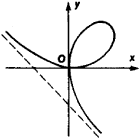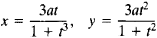# Unicursal Curve

Also found in: Dictionary.
The following article is from The Great Soviet Encyclopedia (1979). It might be outdated or ideologically biased.

## Unicursal Curve

in mathematics, a plane curve that can be parametrized by a pair of rational functions Ф(t) and Ψ(t). The most important theorems on unicursal curves are the assertion that an algebraic curve with the maximal number of double points permitted by its order is unicursal and its converse, that is, the assertion that every unicursal curve is an algebraic curve with the maximal number of double points permitted by its order. Here it is assumed that, as usual, a point of higher multiplicity is counted in terms of its “double point equivalents,” so that, for example, a triple point is equivalent to three double points.Figure 1

The maximal number of double points of an algebraic curve of order n is δ = (n – 1)(n – 2)/2. If the curve of order n has r double points, then the difference δ – r is called its defect, or genus. Therefore, a unicursal curve can be defined as an algebraic curve of genus zero. It is clear that lines and quadratic curves cannot have double points and so are unicursal. A curve of order 3 is unicursal if it has one double point, and a curve of order 4 is unicursal if it has three double points.

Figure 1 represents a third-order curve known as the folium of Descartes. It has one double point and so is unicursal. In fact, the folium of Descartes admits the parametrizationThe parameter t is the tangent of the angle between the x-axis and the radius vector of the point (x,y).

In counting the number of double points, we must not rely on the actual graph of the curve, since we may leave out double points at infinity or complex double points. For example, the fourth-order curve known as the lemniscate of Bernoulli has just one real double point and two circular double points and so is unicursal.

Unicursal curves are important in the theory of integrals of algebraic functions. Every integral of the form

ʃ R(x,y) dx

where R is a rational function of two variables and x and y satisfy an equation F(x,y) = 0, which defines a unicursal curve, can be reduced to an integral of a rational function and expressed in terms of elementary functions.